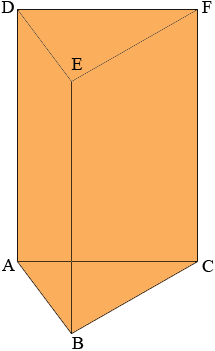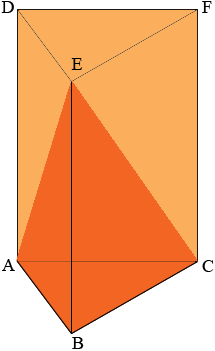SEARCH HOMEMath Central Quandaries & QueriesQuestion from Jeff: If I have a wedge shaped object (ie, a triangle of non-specific type extruded along a length) and it is sliced from the two-corner base at one end to the one-point tip at the other, what is the volume of the upper (smaller) part as a percentage of the whole? I hope I have explained it clearly enough. Thanks.Hi Jeff,

I am going to take the wedge and stand it on its end. The volume of the wedge is the area of the triangle ABC times the height of the wedge, h = |BE|.Next I slice from the line segment AC to the vertex E to form the solid figure ABCE that you described.The solid figure ABCE can be described as follows. Take a triangle ABC in the plane and a point E not in the plane. Join each point on the perimeter of the triangle to the point E to form the boundary of solid ABCE. The volume of the solid ABCE can be found from a surprising geometric theorem.

If you take some region R in the plane with an area, some point V not in the plane and form a cone by joining every point on the boundary of R to V with a straight line then the volume of the cone is
(1/3) × (the area of the base) × (the height).

Thus the volume of the solid ABCE is (1/3) × (area of triangle ABC) × h. Hence the volume of ABCE is one third of the volume of the wedge.

HarleyMath Central is supported by the University of Regina and The Pacific Institute for the Mathematical Sciences.